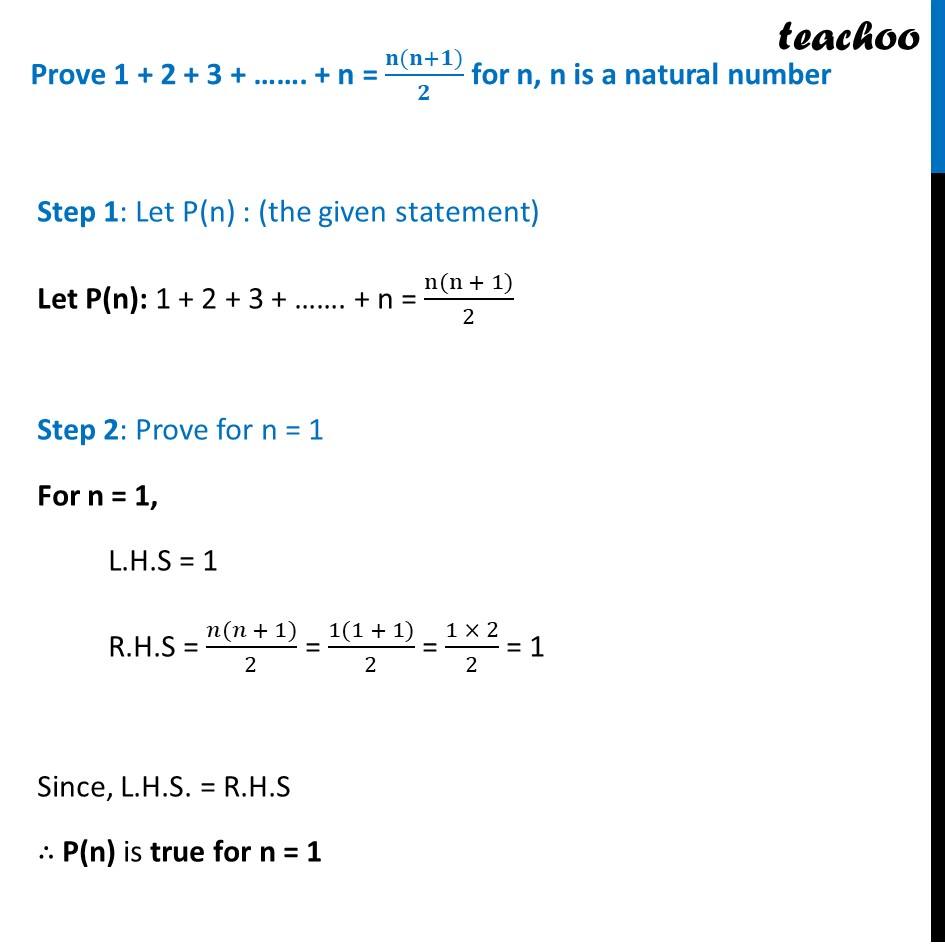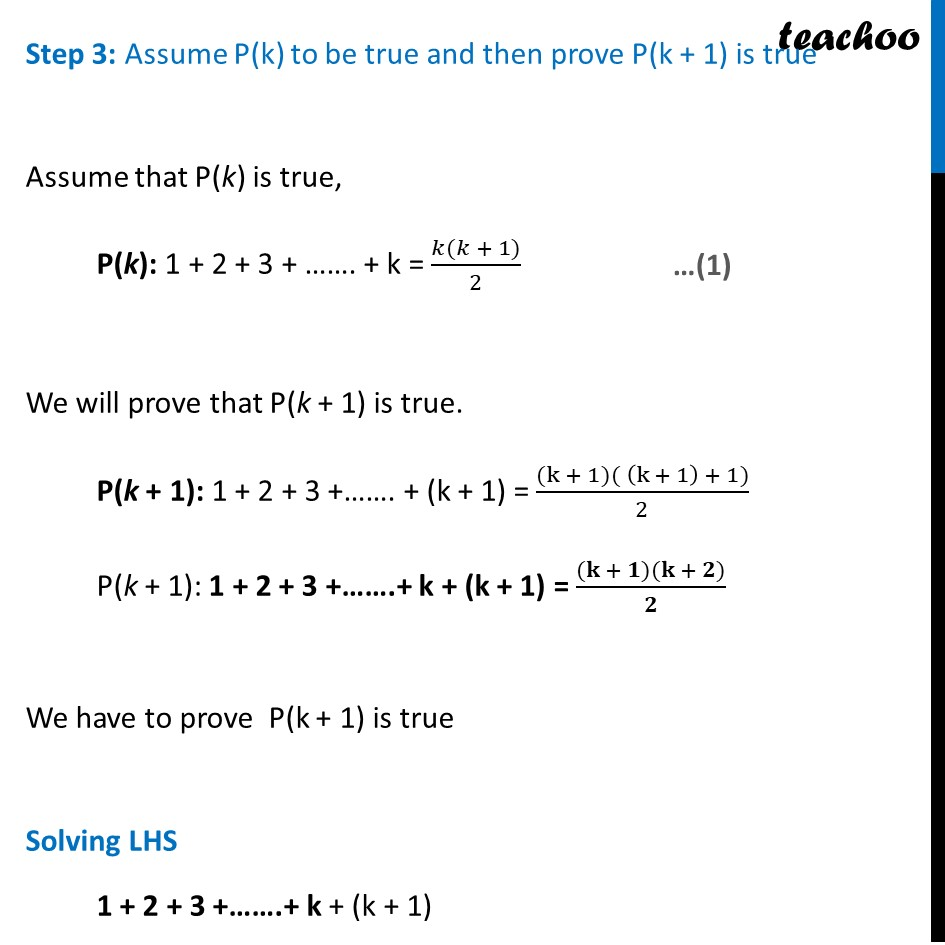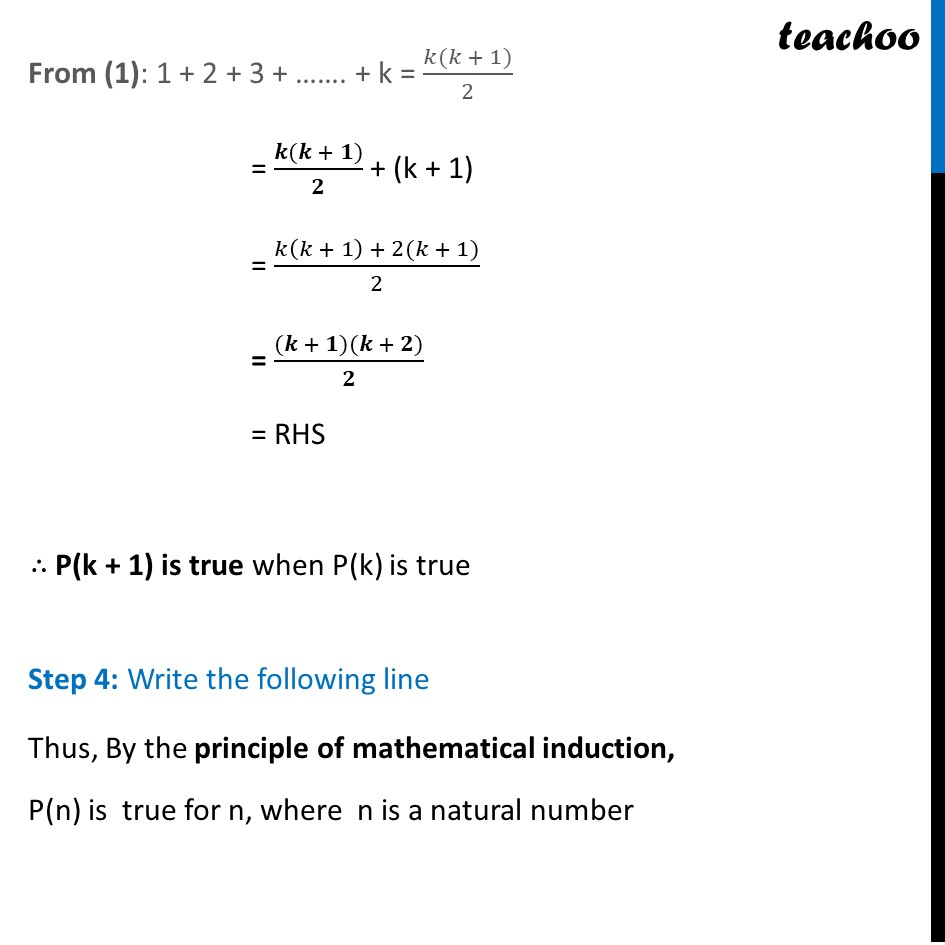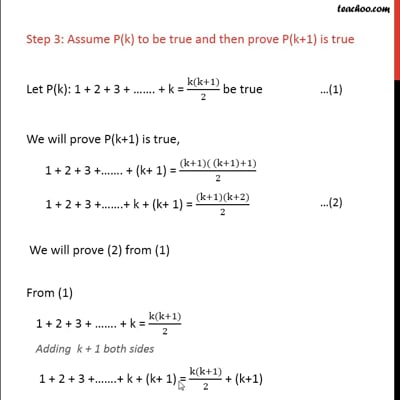Chapter 4 Class 11 Mathematical Induction
Serial order wiseThis video is only available for Teachoo black users

Solve all your doubts with Teachoo Black (new monthly pack available now!)

### Transcript

Prove 1 + 2 + 3 + ……. + n = (𝐧(𝐧+𝟏))/𝟐 for n, n is a natural number Step 1: Let P(n) : (the given statement) Let P(n): 1 + 2 + 3 + ……. + n = (n(n + 1))/2 Step 2: Prove for n = 1 For n = 1, L.H.S = 1 R.H.S = (𝑛(𝑛 + 1))/2 = (1(1 + 1))/2 = (1 × 2)/2 = 1 Since, L.H.S. = R.H.S ∴ P(n) is true for n = 1 Step 3: Assume P(k) to be true and then prove P(k + 1) is true Assume that P(k) is true, P(k): 1 + 2 + 3 + ……. + k = (𝑘(𝑘 + 1))/2 We will prove that P(k + 1) is true. P(k + 1): 1 + 2 + 3 +……. + (k + 1) = ((k + 1)( (k + 1) + 1))/2 P(k + 1): 1 + 2 + 3 +…….+ k + (k + 1) = ((𝐤 + 𝟏)(𝐤 + 𝟐))/𝟐 We have to prove P(k + 1) is true Solving LHS 1 + 2 + 3 +…….+ k + (k + 1) From (1): 1 + 2 + 3 + ……. + k = (𝑘(𝑘 + 1))/2 = (𝒌(𝒌 + 𝟏))/𝟐 + (k + 1) = (𝑘(𝑘 + 1) + 2(𝑘 + 1))/2 = ((𝒌 + 𝟏)(𝒌 + 𝟐))/𝟐 = RHS ∴ P(k + 1) is true when P(k) is true Step 4: Write the following line Thus, By the principle of mathematical induction, P(n) is true for n, where n is a natural number